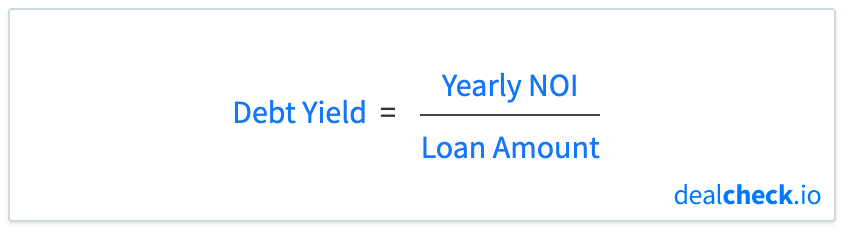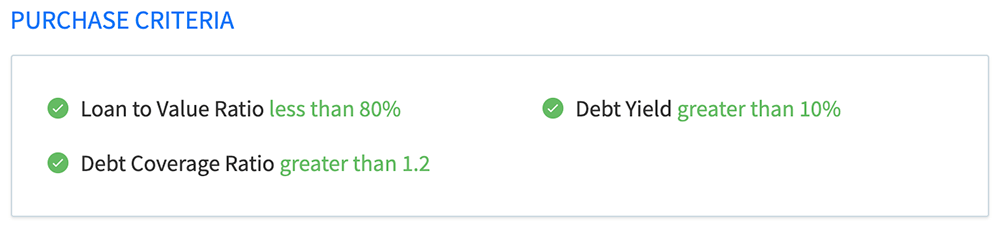The debt yield is an indicator of leverage and loan risk that is often used by real estate lenders when underwriting loans for rental properties, especially when working with commercial real estate.

In simple terms, debt yield can be used to estimate how long it will take for a lender to recover their investment if the borrower were to default on their loan and the lender had to take possession of the property.

While traditionally commercial lenders relied primarily on the debt service coverage (DSCR) and the loan to value (LTV) ratios when underwriting loans, the debt yield has recently been growing in popularity and is used by more and more lenders as an underwriting criteria.

### The Debt Yield Formula

The debt yield can be calculated by dividing a property’s yearly net operating income (NOI) by the total loan amount:A property’s net operating income can be calculated by subtracting all operating expenses from its operating income. In other words, it is the net income a property will generate before accounting for loan payments, depreciation and capital reserves.

A property’s total loan amount is the full amount of the new loan at the time of underwriting.

### How Lenders Use Debt Yield to Assess Underwriting Risk

Suppose Property A has a yearly net operating income (NOI) of \$200,000 and a total loan amount of \$2,000,000. In this case, the debt yield of this property is calculated as \$200,000 / \$2,000,000 = 10%.

Another property (Property B) has the same loan amount (\$2,000,000), but an NOI of \$150,000. Its debt yield would then be \$150,000 / \$2,000,000 = 7.5%.

To a lender, a lower debt yield indicates higher leverage and therefore higher credit risk, while a higher debt yield indicates lower leverage and therefore lower credit risk. Out of the two examples above, Property A would be more attractive to a lender, as its debt yield is higher.

Many lenders have a minimum debt yield that they would consider when underwriting new loans. While the exact criteria will vary by lender, it is common to see 10-12% as the minimum debt yield a lender will accept.

### How to Use Debt Yield to Calculate the Maximum Loan Amount

The debt yield formula shown above can easily be re-arranged to solve for the total loan amount:As the net operating income of a property is usually fixed and not subject to manipulation, and given the fact that most lenders have a minimum debt yield they are willing to accept, this gives them a convenient way to calculate the maximum loan amount for a given property.

Coming back to the Property B example above, if a lender has a minimum debt yield requirement of 10%, then they would calculate the maximum loan amount they would be willing to finance as \$150,000 / 0.1 = \$1,500,000 – quite a bit lower than what was originally proposed.

### Debt Yield vs. Debt Service Coverage and Loan to Value Ratios

Unlike the debt service coverage (DSCR) and loan to value (LTV) ratios, the debt yield provides a measure of credit risk that is less susceptible to manipulation and market conditions.

Specifically, it is independent of the loan interest rate and amortization period, as well as of the property’s market value. It is not possible to inflate the debt yield by adjusting the loan terms or using a more favorable property valuation method.

Consider the loan to value ratio, calculated by dividing the total loan amount by the property’s market value. While the loan amount is fixed, a property’s market value is not only volatile but can potentially be inflated, giving an appearance of a lower LTV and a safer loan scenario than what it actually may be.

A similar situation is possible when looking at the debt service coverage ratio in isolation. DSCR is calculated by dividing a property’s yearly net operating income by the yearly debt service. While this ratio appears similar to debt yield, it is actually easy to change the yearly debt service by adjusting the interest rate of the loan or changing its amortization period. For example, increasing the amortization period would artificially inflate the DSCR, even though the credit risk may remain the same.

Because of this, it is becoming increasingly common for lenders to consider all three measures of underwriting risk – the loan to value ratio, debt service coverage ratio, and debt yield when making underwriting decisions. Lenders will often look at all three ratios and use the least favorable one to calculate the maximum loan amount and credit risk.### Calculate Debt Yield and Dozens of Other Metrics in Seconds

The DealCheck property analysis app makes it easy to calculate the debt yield, along with dozens of other property analysis metrics for both commercial and residential rental properties in seconds.